# Selective Topics on Optimization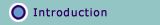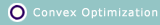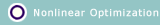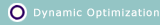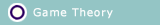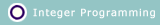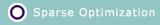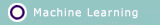- - - - - - - - - - - - Course Information: In this course we will purpose some selected topics in optimization, which included the convex optimization, nonlinear optimization, stochastic optimization, and etc. After taking this course will provide you with the basic techniques to solve optimization problems in your future research and works. This class considers various optimization techniques that can be applied to different scenarios. These techniques will be categorized and then compared for their advantages and disadvantages. Some applications will be given as examples. The topics include but are not limited to Optimization Formulation and Analysis We discuss how to formulate the problem as an optimization issue. Specifically, we study what the objectives are, what the parameters are, what the practical constraints are, and what the optimized performances across the different layers are. The tradeoffs between the different optimization goals and different users' interests are also investigated. The goal is to provide the students a new perspective from the optimization point of view for variety of problems in engineering fields. Mathematical Programming If the optimization problem is to find the best objective function within a constrained feasible region, such a formulation is sometimes called a mathematical program. Many real-world and theoretical problems can be modeled in this general framework. We discuss the four major subfields of the mathematical programming: linear programming, convex programming, nonlinear programming, and dynamic programming. Integer/Combinatorial Optimization The discrete optimization is the problem in which the decision variables assume discrete values from a specified set. The combinatorial optimization problems, on the other hand, are problems of choosing the best combination out of all possible combinations. Most combinatorial problems can be formulated as integer programs. Integer optimization is the process of finding one or more best (optimal) solutions in a well defined discrete problem space. The major difficulty with these problems is that we do not have any optimality conditions to check if a given (feasible) solution is optimal or not. We listed several possible solutions such as relaxation and decomposition, enumeration, knapsack problem and cutting planes. Game Theory Game theory is a branch of applied mathematics that uses models to study interactions with formalized incentive structures (''games"). It studies the mathematical models of conflict and cooperation among intelligent and rational decision makers. Rational means that each individual's decision-making behavior is consistent with the maximization of subjective expected utility. Intelligent means that each individual understands everything about the structure of the situation, including the fact that others are intelligent rational decision makers. We have discussed four different types of games, namely, the non-cooperative game, repeated game, cooperative game, and auction theory. The basic concepts are listed and simple examples are illustrated. Recommended Reading: Zhu Han, Dusit Niyato, Walid Saad, Tamer Basar, and Are Hjorungnes,Game Theory in Wireless and Communication Networks: Theory, Models and Applications, Cambridge University Press, UK, 2011. Steven Boyd's videos for convex optimization, lecture notes Handout for parts of book. Zhu Han and K. J. Ray Liu, Resource Allocation for Wireless Networks: Basics, Techniques, and Applications, Cambridge University Press, 2008. Introduction, ppt Notice for the web-viewers outside UH: You are welcomed to use the slides but please let me know if there are any error and any way for improvement. - - - - - - - - - - - -